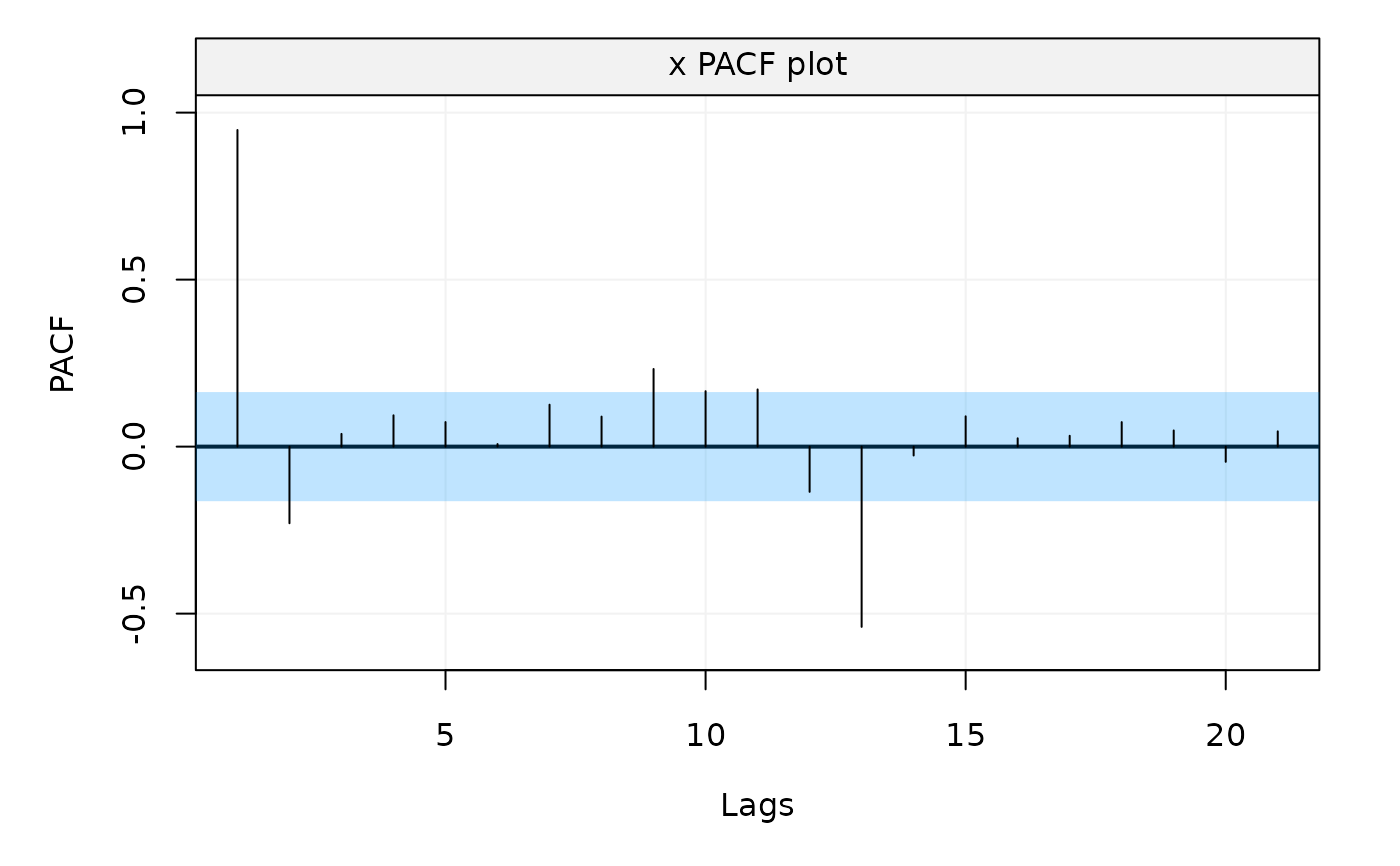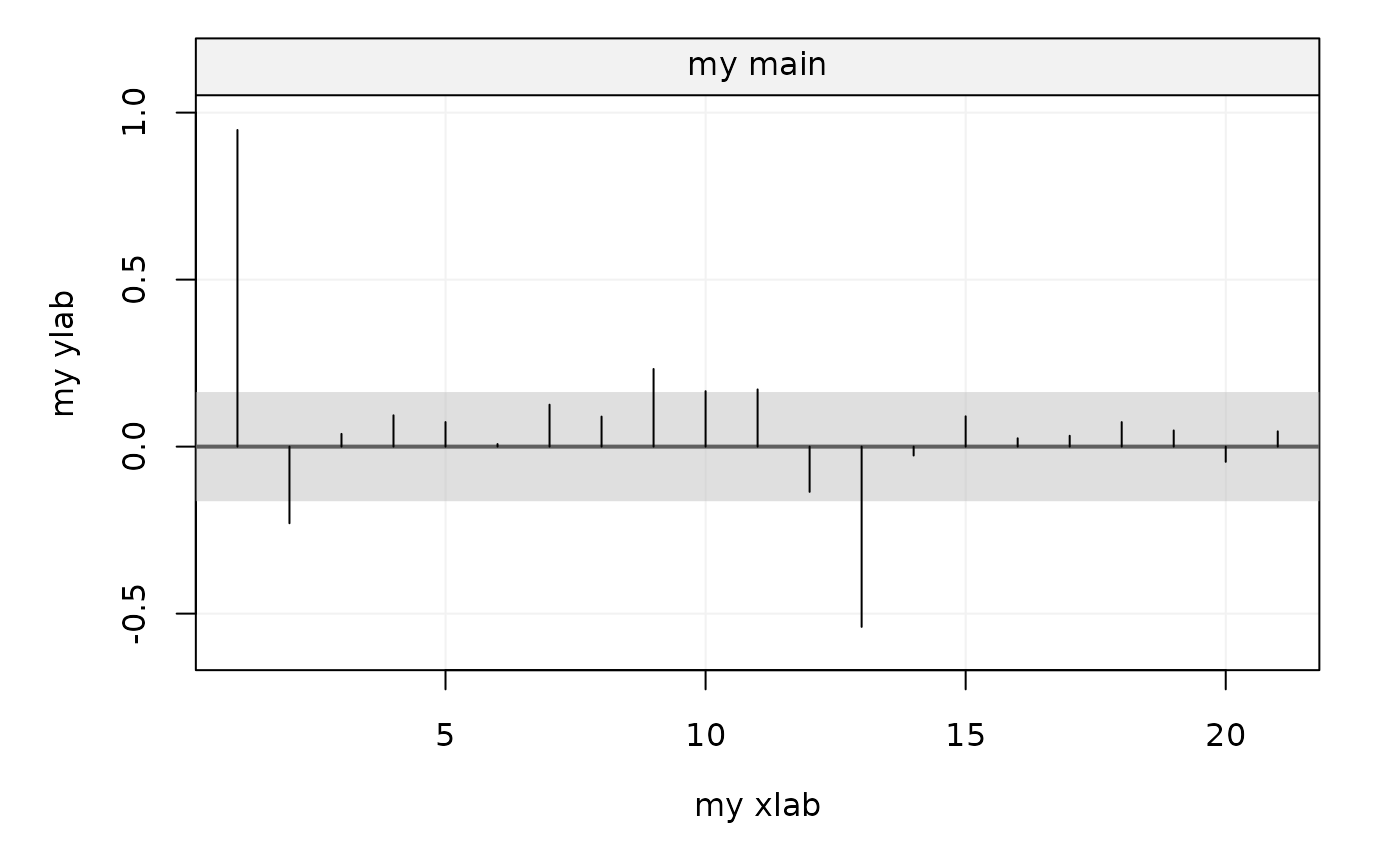The function plots the output of the theo_pacf and auto_corr functions (partial autocovariance or autocorrelation functions).

# S3 method for PACF
plot(
x,
xlab = NULL,
ylab = NULL,
show.ci = TRUE,
alpha = NULL,
col_ci = NULL,
transparency = NULL,
main = NULL,
parValue = NULL,
...
)

## Arguments

x

A "PACF" object output from theo_pacf or auto_corr.

xlab

A string indicating the label of the x axis: the default name is 'Lags'.

ylab

A string indicating the label of the y axis: the default name is 'PACF'.

show.ci

A bool indicating whether to show the confidence region. Defaults to TRUE.

alpha

A double indicating the level of significance for the confidence interval. By default alpha = 0.05 which gives a 1 - alpha = 0.95 confidence interval.

col_ci

A string that specifies the color of the region covered by the confidence intervals (confidence region).

transparency

A double between 0 and 1 indicating the transparency level of the color defined in col_ci. Defaults to 0.25.

main

A string indicating the title of the plot. Default name is "Variable name PACF plot'.

parValue

A vector defining the margins for the plot.

...

## Author

Yunxiang Zhang and Yuming Zhang

## Examples

# Plot the Partial Autocorrelation
m = auto_corr(datasets::AirPassengers, pacf = TRUE)
plot(m)# More customized CI
plot(m, xlab = "my xlab", ylab = "my ylab", show.ci = TRUE,
alpha = NULL, col_ci = "grey", transparency = 0.5, main = "my main")